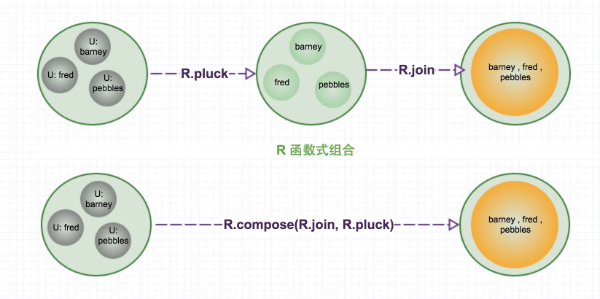# Ramdajs函数编程``````var users = [
{ 'user': 'barney',  'age': 36 },
{ 'user': 'fred',    'age': 40 },
{ 'user': 'pebbles', 'age': 18 }
];

var names = _.chain(users)
.pluck('user')
.join(" , ")
.value();
console.log(names);
``````

ramdajs是一个更具有函数式代表的JavaScript库，可以在这里了解更多关于它的信息http://ramdajs.com/0.17/。它的这种能力主要来自它自有的两大能力：自动柯里化和函数参数优先于数据。

#### 自动柯里化

ramdajs利用这一技术，默认所有API函数都支持自动柯里化。这为它提供了可以将另一个函数组合的先决条件。如常用的map操作需要接受两个参数，在ramdajs中可以如下两种方式实现：

``````R.map(function(item){
return item *2;
},
[2,3,5]
); //输出[4, 6, 10]

var map = R.map(function(item){
return item *2;
});
map([2,3,5]); //输出[4, 6, 10]
``````

#### 函数参数优先于数据

1. R.multiply(a, b)：实现 a *b； 2：R.map(func, data)：实现集合 a –> b的map。

``````R.multiply(10, 2); // 20

R.multiply(10) (2); // 20
``````

``````R.map(R.multiply(2)) ([2, 5, 10, 80]); // [4, 10, 20, 160]
``````

R.map(R.multiply(2))的返回值也是一个函数，它是一个组合转换函数。它组合了map和multiply行为。它利用R.map组合封装了R.multiply(2)返回的柯里化函数，它等待map函数传入对应的被乘数。

#### ramdajs的组合

1. R.pluck(prop)：选择对象固定属性；
2. R.join(data)：对数组的字符串拼接。

``````var joinUserName = R.compose(R.join(" , "), R.pluck("user"));
joinUserName(users); // "barney , fred , pebbles"
````````````var joinUserAge = R.compose(R.join(" , "), R.pluck("age"));
joinUserAge(users); // "36 , 40 , 18"
``````

1. R.subtract(a, b)：实现 a – b 数学算法。

``````var joinUserBrithDay = R.compose(R.join(","),R.map(R.subtract(new Date().getFullYear())),R.pluck("age"));
joinUserBrithDay(users); // "1979,1975,1997"
``````

lodash实现：

``````_.chain(users)
.sortBy("age")
.first()
.value();
``````

``````var youngestUser = R.compose(R.head, R.sortBy(R.prop("age")));
youngestUser(users); // Object {user: "pebbles", age: 18}
``````

``````var olderUser = R.compose(R.head, R.reverse, R.sortBy(R.prop("age")));
olderUser(users); // Object {user: "fred", age: 40}
``````